# College Chemistry : Strong and Weak Acids/Bases

## Example Questions

### Example Question #1 : Strong And Weak Acids/Bases

Which of the following is a weak base?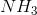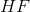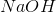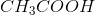Explanation:

Strong acids and bases are those that ionize completely in solution. In contrast, weak acids and bases ionize only partially in solution., hydrogen fluoride, is an example of a very strong acid. In solution, it dissolves completely to form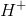and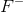, sodium hydroxide, is an example of a very strong base. In solution, it dissolves completely to form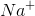and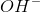., acetic acid, is an example of a weak acid. In solution, it dissolves partially to form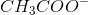and., ammonia, is an example of a weak base. In solution, it accepts a proton to form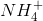and.

### Example Question #2 : Strong And Weak Acids/Bases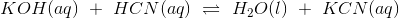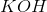reacts with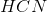in aqueous solution according to the chemical equation provided. If it took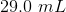of a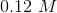solution to titrate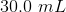of ansolution, what was the concentration of originalsolution?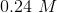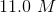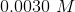Explanation:

Using the concentration ofas a conversion factor, we can convert the volume ofto moles by dimensional analysis: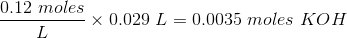At the equivalence point of the titration: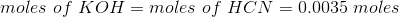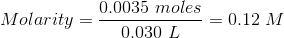### Example Question #3 : Strong And Weak Acids/Bases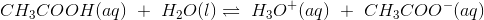Determine the acid dissociation constant expression for the given reaction.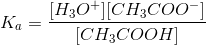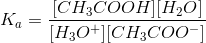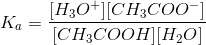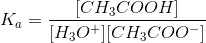Acid dissociation constant which is denoted as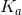is the equilibrium constant for the ionization of an acid. Therefore, the numerator contains the product of the concentrations of the substances on the product side of the chemical equation. The denominator contains the product of the concentrations of the substances on the reactant side of the chemical equation.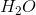is omitted in the acid dissociation constant expression because as the solvent it is in excess and therefore the change in its concentration is negligible in comparison to the other substances in solution.